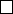7Transformation groups

IA Vectors and Matrices7.2 Length preserving matrices
Theorem. Let P O(n). Then the following are equivalent:
(i) P is orthogonal
(ii) |P x| = |x|
(iii) (P x)
T
(P y) = x
T
y, i.e. (P x) · (Py) = x ·y.
(iv) If (v
1
, v
2
, ··· , v
n
) are orthonormal, so are (P v
1
, P v
2
, ··· , P v
n
)
(v) The columns of P are orthonormal.
Proof. We do them one by one:
(i) (ii): |P x|
2
= (P x)
T
(P x) = x
T
P
T
P x = x
T
x = |x|
2
(ii) (iii): |P (x + y)|
2
= |x + y|
2
. The right hand side is
(x
T
+ y
T
)(x + y) = x
T
x + y
T
y + y
T
x + x
T
y = |x|
2
+ |y|
2
+ 2x
T
y.
Similarly, the left hand side is
|P x + P y|
2
= |P x|
2
+ |P y| + 2(P x)
T
P y = |x|
2
+ |y|
2
+ 2(P x)
T
P y.
So (P x)
T
P y = x
T
y.
(iii) (iv): (P v
i
)
T
P v
j
= v
T
i
v
j
= δ
ij
. So P v
i
’s are also orthonormal.
(iv)
(v): Take the
v
i
’s to be the standard basis. So the columns of
P
, being
P e
i
, are orthonormal.
(v)
(i): The columns of
P
are orthonormal. Then (
P P
T
)
ij
=
P
ik
P
jk
=
(P
i
) · (P
j
) = δ
ij
, viewing P
i
as the ith column of P . So P P
T
= I.
Therefore the set of length-preserving matrices is precisely O(n).